# Test: Percentages- 2

## 20 Questions MCQ Test Quantitative Reasoning for GMAT | Test: Percentages- 2

Description
Attempt Test: Percentages- 2 | 20 questions in 40 minutes | Mock test for GMAT preparation | Free important questions MCQ to study Quantitative Reasoning for GMAT for GMAT Exam | Download free PDF with solutions
QUESTION: 1

### A toy manufacturer runs his plant for 30 days in a month such that his daily output is constant during the month. He undertakes the maintenance of his plant every 6 months for 5 days in a month, during which period no toys are produced. As he has to undertake the maintenance of the plant this month, he increases his constant daily output of toys by 10 percent compared to the last month’s constant daily output. If the manufacturer wants to keep his monthly revenue unchanged as compared to last month, by approximately what percentage should be increase the price per toy?

Solution:

Given:

Earlier Month

• Total running duration of the plant in the month = 30 days
• Let the daily output during the month = n toys
• Let the total monthly revenue be P

This Month

• Maintenance Period during the month= 5 days
• Total running duration of the plant in the month = 30 - 5 = 25 days
•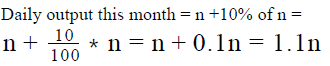•  Let the total monthly revenue be Q

To Find: To keep P = Q, by what percentage should the manufacturer increase the price per toy?

Approach:

1. The percentage increase in the selling price needed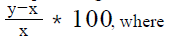• x is the selling price per toy in the Earlier Month
• And, y is the selling price per toy in This Month
2. Calculating monthly revenue of the Earlier Month (P)
• Number of toys produced in 1 day = n
• Total number of days = 30
• Therefore, total number of toys produced in the Earlier Month = 30n
•  As assumed above, the selling price per toy be x
• Hence, monthly revenue P = (30n) * x = 30nx
3. Calculating monthly revenue of This Month(Q)
• Number of toys produced in 1 day = 1.1n
• Total number of days = 25
• Therefore, total number of toys produced This Month = 1.1n*25
• As assumed above, the selling price per toy be y
• Hence, monthly revenue Q = (1.1n * 25) * y = 1.1*25ny

Working out:

1. Equating the monthly revenue of both the months, we have

​​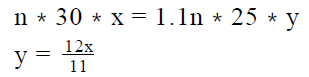2. So, the percentage increase in the price can be calculated as: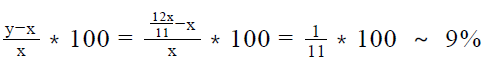QUESTION: 2

### If b is a prime number, what percent of 3 is b? (1) b is more than 200% greater than 2 and less than 40% of 32  (2) 3b/7  is 40% less than 5

Solution:

Step 1 & 2: Understand Question and Draw Inference

Given: Prime number b
To find: What percent of 3 is b?
Let b be x percent of 3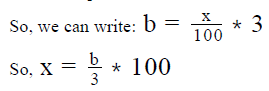Therefore, in order to find the value of x, we need to find the value of b

Step 3 : Analyze Statement 1 independent

Statement 1 says that ‘b is more than 200% greater than 2 and less than 40% of 32’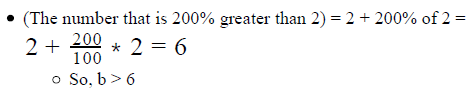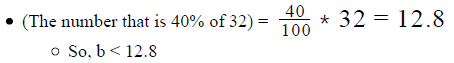• Therefore, as per Statement 1, b is a prime number that lies between 6 and 12.8, exclusive
• So, possible values of b = {7, 11}
• Since Statement 1 doesn’t lead to a unique value of b, it is not sufficient

Step 4 : Analyze Statement 2 independent

Statement 2 says that ‘ 3b/7  is 40% less than 5'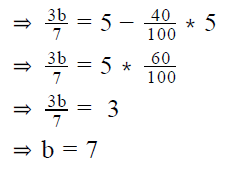So, b = 7
Since Statement 2 leads to a unique value of b, it is sufficient to answer the question

Step 5: Analyze Both Statements Together (if needed)

Since we get a unique answer in Step 4, this step is not required

QUESTION: 3

### The population of city X increased by 10% in year 2013 and 15% in year 2014. What is the approximate population of the city at the end of year 2014, if population of the city at the starting of year 2013 was 21,000?

Solution:

Given:

• Population of city X at starting of the year 2013 (Ps2013 ) = 21,000
• Percentage increment in population of city X in year 2013(P.I2013 )= 10%
• Percentage increment in population of city X in year 2014(P.I2014 ) = 15%

To Find :

• Population of the city at end of year 2014 (Pe2014 )

Approach:

• In order to find the Population of the city at end of the year 2014 (Pe2014 ), we need to first work out the population at the start of the year 2014 (Ps2014).

For calculating the population at start of year 2014, we will find the population of the city at end of year 2013 (Pe2013 ), which is equal to the population at the start of the year 2014.

• We know that → (Ps2013 ) = 21,000 & (P.I. 2013)=10%
• So we can find the population of the city at end of year 2013 (Pe2013 ) or at the starting of the year 2014 (Ps2014 ) by → (Ps2014 )= Ps2013 + (P.I.2013 )* (Ps2013 )
• Having calculated the population of the city X at the start of year 2014 and using the percentage increment of the year 2014, we will be able to calculate the population at end of year 2014 by → (Pe2014)= Ps2014 +(P.I.2014 )* (Ps2014 )

Working out: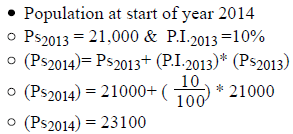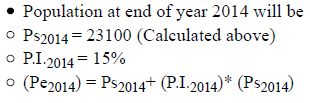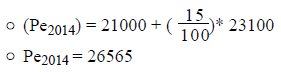So population at end of year 2014 will be 26565, which is approximately equal to 26500.
Hence answer option E is correct

QUESTION: 4

The price to earnings ratio of the pharmaceutical industry on a stock exchange was p at the beginning of the year 2014 and q at the end of the year 2014. Company X, which was a pharmaceutical company had its earnings to price ratio equal to a at the beginning of the year 2014 and b at the end of the year 2014. By what percentage was the percentage increase in the price to earnings ratio of company X less than that of the pharmaceutical industry?

Solution:

Given:

• Pharmaceutical Industry
• Price to Earnings ratio at the beginning of the year 2014 = p
• Price to Earnings ratio at the end of the year 2014 = q
• Company X
• Earnings to Price ratio at the beginning of the year 2014 = a
• Price to Earnings ratio at the beginning of the year 2014 = 1/a
• Earnings to Price ratio at the end of the year 2014 = b
• Price to Earnings ratio at the end of the year 2014 = 1/b

To Find: By what % was the % increase in the price to earnings ratio of company X less than that of the pharmaceutical industry?

Approach:

1. Calculate the increase in the price to earnings ratio of the pharmaceutical industry using

Increase in the ratio of the industry = price to earnings ratio in the beginning of the year 2014 – price to earnings ratio at the end of the year 2014

2. Calculate the percentage increase (Pi ) in the price to earnings ratio using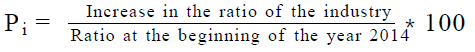3. Calculate the increase in the price to earnings ratio of the company X using Increase in the ratio of company X = price to earnings ratio in the beginning of the year 2014 – price to earnings ratio at the end of the year 2014

4. Calculate the percentage increase(Xi ) in the price to earnings ratio using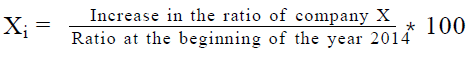5. Calculate the percentage of percentage increase of the difference of P and X using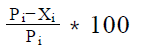Working out: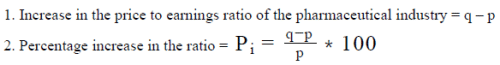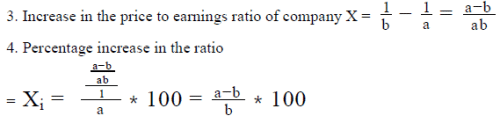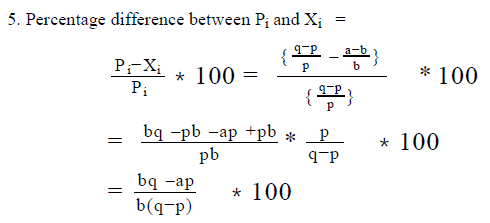QUESTION: 5

An airline allowed free baggage only up to 20 kilograms of weight. Each extra kilogram of weight cost a passenger \$5 per kilogram. Of the total weight of the baggage that Roger was carrying, 60 percent of the weight was more than the free baggage allowance. If the total ticket cost of Roger including the excess baggage fare was \$600, by what percentage did his ticket cost increase because of the excess baggage that he was carrying?

Solution:

Given:

• Free baggage allowed = 20 kilograms
• Cost of each kilogram above 20 kilograms = \$5
• Let the total baggage carried by Roger be x kilograms
• Excess baggage carried by Roger = 60% of x = 0.6x Kilograms
• Total ticket cost including the excess baggage fare = \$600

To Find: by what percentage did his ticket cost increase because of the excess baggage that he was carrying?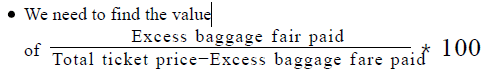Approach:

1. We need to first find the fare paid by Roger because of the excess baggage he was carrying.
• We know that out of his total baggage, 60% was above 20 kilograms. That tells us that the rest 40% of the baggage was equal to 20 kilograms.
• Using the relation above, we can find the excess baggage carried by Roger and thus the fare paid by him because of the excess baggage.
• Knowing that each extra kilogram of baggage cost \$5, we can calculate the fare paid by him because of the excess baggage

Working out:

1. So, we know that 40% of x = 20 kilograms
• Thus x = 50 kilograms. Hence, Roger was carrying 50 – 20 = 30 kilograms of excess baggage
• Excess fare paid by Roger because of the baggage = \$(30 * 5) = \$150
2. Total fare excluding the fare paid for the baggage = \$(600 – 150) = \$450
3. Thus, Roger’ ticket fare increased by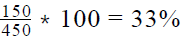because of the excess baggage that he was carrying.

QUESTION: 6

An internet-based company that came into business on January 1, 2015 acquired 250 customers in the first month. If the company
had 20% month-on-month growth for the next 2 months and 10% month-on-month growth after that, how many customers did the
company have on May 1, 2015?

Solution:

Given:

• January: 250 customers
• February: 20% month- on – month growth
• March: 20% month – on – month growth
• April: 10% month – on – month growth

To Find: Number of customers on May 1

Approach:

1. Number of customers on May 1 = Number of customers on Apr 1 + Growth in Apr
• Growth in Apr = (Growth Rate in Apr)*(Customers on Apr 1)
• So, Number of customers on May 1 = (1 + Growth Rate in Apr)*(Number of customers on Apr 1)
2. Similarly, Number of customers on Apr 1 = (1 + Growth Rate in Mar)*(Number of customers on Mar 1)
3. Number of customers on Mar 1 = (1 + Growth Rate in Feb)*(Number of customers on Feb 1)
4. Number of customers on Feb 1 = Number of customers acquired during Jan

Working out: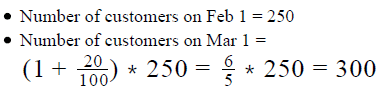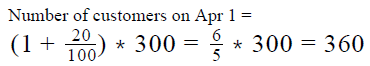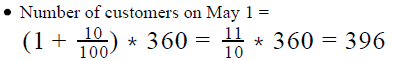Looking at the answer choices, we see that Option B is the correct answer

QUESTION: 7

If x and y are positive numbers, x is what percent of y?
(1) 3x is 72% of 2y
(2) y = 2x + 1

Solution:

Step 1 & 2: Understand Question and Draw Inference

Given: x, y > 0
To find: x is what percent of y?

• Let x be z percent of y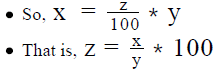• So, in order to answer the question, we need to know the ratio x / y

Step 3 : Analyze Statement 1 independent

Statement 1 says that ‘3x is 72% of 2y’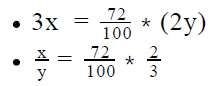• Thus, Statement 1 leads us to a unique value of the ratio x/ y
• So, Statement 1 alone is sufficient

Step 4 : Analyze Statement 2 independent

Statement 2 says that ‘y = 2x + 1’
Dividing both sides of the above equation by y: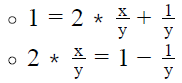• The value of  x/y in the above equation will depend on the value of y
• Since we do not know the value of y, we cannot find a unique value of the ratio x/y
• So, Statement 2 alone is not sufficient to answer the question

Step 5: Analyze Both Statements Together (if needed)

Since we’ve already arrived at a unique answer in Step 3, this step is not required

QUESTION: 8

An army’s recruitment process included n rounds of selection tasks. For the first a rounds, the rejection percentage was 60 percent per round. For the next b rounds, the rejection percentage was 50 percent per round and for the remaining rounds, the selection percentage was 70 percent per round. If there were 100000 people who applied for the army and 1400 were finally selected, what was the value of n?

Solution:

Given:

• Total number of applicants = 100000
• Total people selected = 1400
• Total number of rounds = n
• Rejection percentage of each round for the first a rounds = 60%
• Selection percentage of each round for the first n rounds = 40%
• Rejection percentage of each round for the next b rounds = 50%
• Selection percentage of each round for the next b rounds = 50%
• Selection percentage of each round for the remaining rounds(let’s say c rounds) = 70%

To Find: Value of n?

• n = a + b + c
• So, we need to find the values of either all of a, b or c or the value of sum of a , b and c.

Approach:

1. As we know the number of applicants, the selection percentage of each round and the number of people finally selected, we would need to devise an expression for people finally selected in terms of a, b and c.
2. So, number of people who cleared the 1st round = Total number of applicants  x selection percentage of first round
• Similarly, people who cleared the first 2 rounds = Total number of applicants x selection percentage of first
round x selection percentage of second round
• So, people who cleared the first a rounds = Total number of applicants x selection percentage of first round  x selection percentage of second round x  ...selection percentage of a round
• As the selection percentage of each of the first a rounds is the same, we can write, people who cleared the first a rounds = Total number of applicants  x (selection percentage of each round till the a rounds)a
3. Similarly, number of people who cleared the next b rounds as well= Total number of applicants x  (selection percentage of each round till a rounds)x selection percentage of each round till the next b rounds)b
4. So, number of people who cleared the last c rounds as well= Total number of applicants x  (selection percentage of each round till a rounds)x (selection percentage of each round till next b rounds)x  (selection percentage of each round till the last c rounds)c
5. (People who were finally selected) = (people who cleared all the rounds).
6. Now, we will prime factorize the expression on both the sides and equate the exponents of the individual prime factors to find the values of a, b and c.

Working out:

1. Total number of applicants = 100000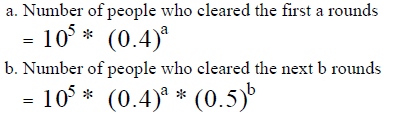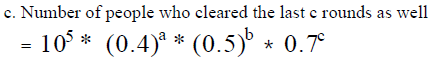2. Number of people who were selected = (Number of people who cleared all the rounds). So, we can write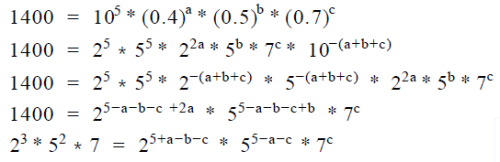3. Comparing the powers of each prime factor on the two sides of the above equation:

• Comparing powers of 7, we have c = 1
• Comparing powers of 5, we have 5 – a –c = 2. As c = 1, we have a = 2
• Comparing powers of 2, we have 5 + a – b – c = 3.
• As c = 1 and a = 2, we have b = 3

4.  So, n = a+ b+ c = 2 + 3+1 = 6

QUESTION: 9

A company had 1000 employees at the beginning of the year 2011. If p percent of the employees present at the beginning of the year left the company during the year and the number of employees at the end of the year was r percent greater than the number of employees at the beginning of the year, how many employees joined the company during the year 2011?

(1) p + r = 30
(2) Had the number of employees leaving the company been r percent of the employees at the beginning of the year and the number of employees at the end of the year been p percent greater than the number of employees at the beginning of the year, the number of employees who joined the company would have remained the same.

Solution:

Step 1 & 2: Understand Question and Draw Inference

• Employees at the beginning of the year 2011 = 1000
• Employees who left during the year 2011 = p% of 1000 =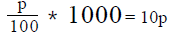• Employees at the end of the year 2011 = 1000 + r% of 1000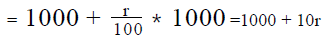• (Employees at the end of 2011) = (Employees at the beginning of the year
•  Employees who left during the year) + (Employees who joined the company during the year 2011)
• Employees who joined the company during the year 2011 = Employees at the end of the year – (Employees at the beginning of the year – Employees who left during the year)

= 1000 + 10r – (1000 -10p) = 10(r + p)

• Therefore to answer the question, we:
• Need to find values of r and p or
• Need to find values of r + p

Step 3 : Analyze Statement 1 independent

1. p + r = 30
Value of r + p is given, hence sufficient to answer.

Step 4 : Analyze Statement 2 independent

1. Had the number of employees leaving the company been r percent of the employees at the beginning of the year and the number of employees at the end of the year been p percent greater than the number of employees at the beginning of the year, the number of employees who joined the company would have remained the same.
• Employees who left the company during the year 2011 = r% of 1000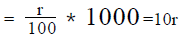• Employees at the end of the year 2011 = 1000 + p% of 1000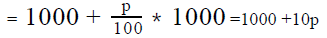Employees joining the company during the year 2011 = (Employees at the end of the year) – (Employees at the beginning of the year – Employees who left during the year)
= (1000 + 10p) – (1000 – 10r)
= 10 (p+ r), which is the same as the expression derived in Step-I.
As we do not have the value of r and p or r + p, the statement is insufficient to answer the question.

Step 5: Analyze Both Statements Together (if needed)

Since we have a unique answer from step 3, this step is not required.

QUESTION: 10

The price of a consumer good increased by p% during 2012 and decreased by 12% during 2013. If no other change took place in
the price of the good and the price of the good at the end of 2013 was 10% higher than the price at the beginning of 2012, what was
the value of p?

Solution:

Given: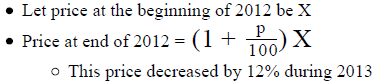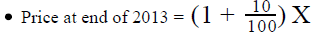To Find: Value of p

Approach:

1. To find the value of p, we need to develop an equation in terms of p
2. We are given 2 relations for the Price at end of 2013:
• Price at end of 2013 = 12% less than the price at end of 2012
• Price at end of 2013 =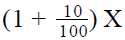3. Using these 2 relations together, we’ll get an equation in p, upon solving which, we’ll get the value of p

Working out: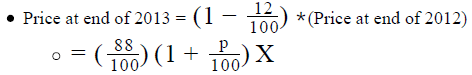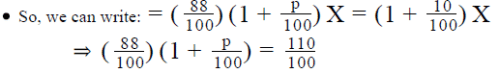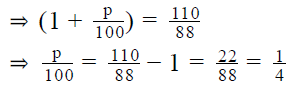• So, p = 25%

Looking at the answer choices, we see that Option D is the correct option

QUESTION: 11

A soccer team played 25% more matches year-on-year in the second year of its formation. It won 84% of the matches it played in the second year, which was an improvement of 5% over the percentage of matches won in the first year. In the third year, the team played 4% fewer matches year-on-year but lost only 12.5% of the matches it played, which was one less than the number of matches lost by the team in the second year. Which of the following statements must be correct?

1. The team lost the same number of matches in the first yea  and the second year
2. The team won the same number of matches in the second year and the third year
3. The team played five more matches in the third year than the first year
Solution:

Given: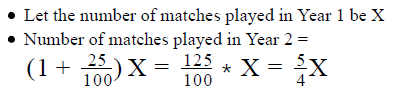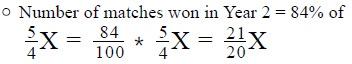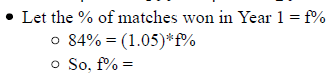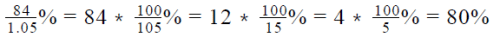• So, in Year 1, the number of matches won = 80% of (Number of matches played in Year 1)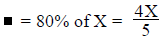• Number of matches played in Year 3 = 4% less than the number of matches played in Year 2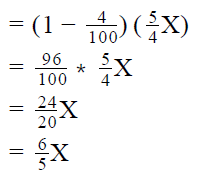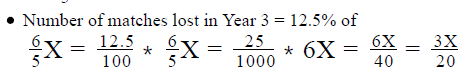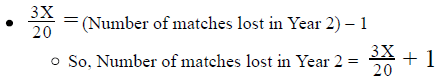To Find: Which of the 3 statements are correct?

Approach:

1. In order to answer the question, we need to know the values (either the exact values or the values in terms of X) for the number of matches Played, Won and Lost in all the 3 years.
2. To make sense of the given information, we’ll represent it visually and then work towards calculating the values that we do not yet know.

Working out:

• Representing the given information visually
• We can represent the given (and inferred) information discussed above in a table as under: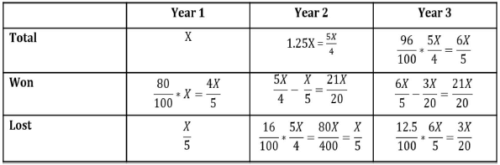• As we see, there are currently 3 unknown values in the table above.
• Finding the unknown values
• Number of matches Lost in Year 1 = (Total matches played in Year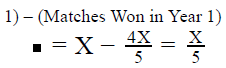• Number of matches Lost in Year 2 = (Total matches played in Year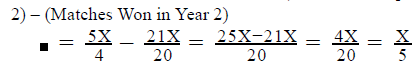• Number of matches Won in Year 3 = (Total matches played in Year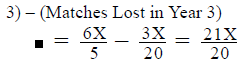• So, the complete table will look as under: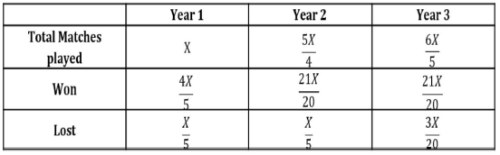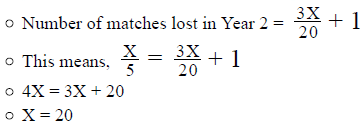• So, after substituting X = 20, the values in the table will look as under: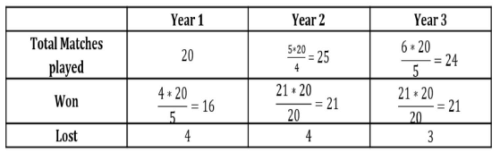• Evaluating the 3 statements
• St-I: The team lost the same number of matches in the first year and the second year
• Statement I is correct
• St-II: The team won the same number of matches in the second year and the third year
• Statement II is also correct
• St-III: The team played five more matches in the third year than the first year
• Statement III is not correct because the team played four more matches in Year 3 than Year 1, not five more matches

Looking at the answer choices, we see that the correct answer is Option E

QUESTION: 12

Daniel was going to purchase an electronic item from a brickand- mortar retail store that offered a discount of 10% on the marked price of the item. Sometime before the purchase, however, he discovered that the same item was also available on an ecommerce website. Though the customers of the ecommerce website had to bear overhead costs of 10% over the marked price, including taxes and delivery charges, the marked price of the item at the retail store was 25% higher than the website. If Daniel wanted to minimize his expense on the item, through which of the two channels – brick-and-mortar retail store and ecommerce website – should he have bought the item and what would have been his percent savings with respect to the other channel?

Solution:

Given:

• Ecommerce website:
• Let Marked price be M
• Retail Store: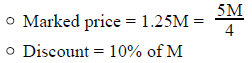To Find: Which channel is less expensive and % savings with respect to the other channel?

Approach:

1. To compare the 2 channels, we need to know the Price that Daniel would pay in each channel
2.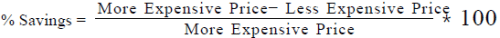Working out: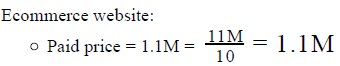• Retail Store:
• % Discount = 10% (of Marked Price)
• So, Discount = 0.1(Marked Price)
• Paid Price = 0.9(Marked Price)=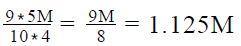• Thus, the paid price is lesser in Ecommerce website.
• % Savings with respect to the other channel =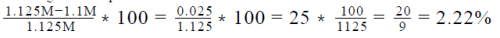Looking at the answer choices, we see that the correct answer is Option D

QUESTION: 13

In a class of 100 students, the percentage of boys in the class is equal to the percentage of students who pass in a test. If 2 out of
every 3 girls in the class pass the test and the number of boys who do not pass the test is 10 less than the total number of girls in the
class, which of the following is the closest to the percentage of boys who passed the test?

Solution:

Given:

• Total students = 100
• Let the number of boys be B
• So, the number of girls = 100 – B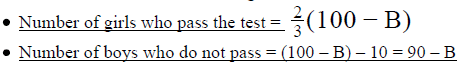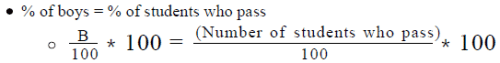• So, number of students who pass = B
• The number of students who don’t pass = 100 – B

To Find: The approximate % of boys who pass the test

Approach:

1.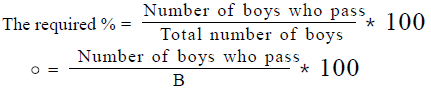2. So, to answer the question, we’ll need:
• Either the values of ‘Number of boys who pass’ and B
• Or the ratio of ‘Number of boys who pass’ and B
3. We’re given information about the 100 students on 2 parameters –
• Gender (Boy, Girl)
• Test Performance (Pass, Don’t Pass)
• So, to be able to work with the information effectively, we will represent the information visually.
4. Then, we’ll work towards finding the required values.

Working out:

Representing the given information visually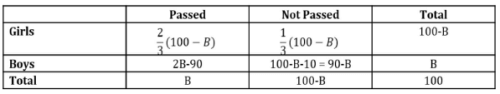• Working towards the required values
• As a first step, let’s complete the empty cells in the above table:
• Girls who don’t pass = (Total number of girls) – (Girls who pass)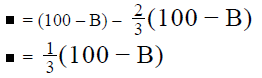• Boys who Pass = (Total number of boys) – (Boys who don’t pass)
• = B – (90 – B) = 2B – 90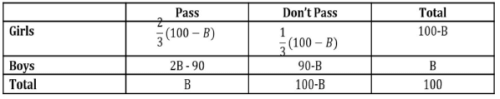• From the above table, we can draft the following linear equation in terms of B:
• (Girls who pass) + (Boys who pass) = Total number of students who pass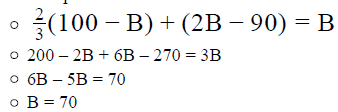• And, Number of boys who pass = 2B - 90 = 2*70 – 90 = 140 – 90 = 50
• So, the Required percentage =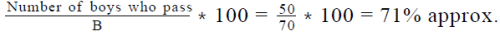Looking at the answer choices, we see that the option that is closest to the required percentage is Option D

QUESTION: 14

The temperature of a place at noon on a certain day is 25% higher than the temperature of the same place at midnight on the same
day. What the temperature of the place at midnight?
(1) The temperature of the place at midnight is 20% lower than the temperature of the same place at noon
(2) The temperature of the place at noon is 10 units greater than the temperature of the place at midnight

Solution:

Step 1 & 2: Understand Question and Draw Inference

Let temperature at midnight be M
And, temperature at noon be N
Given: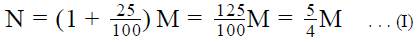We need to find the value of M.
We already have 1 equation between N and M. If we have one more equation between N and M, we can find a unique value of M.

Step 3 : Analyze Statement 1 independent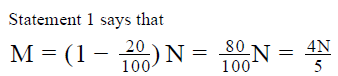This equation restates what we already know from Equation (I). So, not sufficient.

Step 4 : Analyze Statement 2 independent

Statement 2 says that
N = M + 10 . . . (II)
We now have one more equation between N and M. So, Equations (I) and (II) are sufficient to find a unique value of M.

Step 5: Analyze Both Statements Together (if needed)

Since we arrive at a unique answer in Step 4, this step is not required

QUESTION: 15

A wedding decorator receives two sets of light bulbs- A and B. Set A consists of 100 light bulbs of which 20 percent are defective and set B consists of 80 light bulbs of which 50 percent are defective. If the light bulbs from the two sets are mixed, what is the minimum percentage of light bulbs the decorator needs to take out to make sure that the remaining light bulbs are non-defective?

Solution:

Given:

• Set A
• Number of light bulbs = 100
• Defective light bulbs = 20% of 100 = 20
• Non-defective light bulbs = 100 – 20 = 80
• Set B
• Number of light bulbs = 80
• Defective light bulbs = 50% of 80 = 40
• Non-defective light bulbs = 80 – 40 = 40
• Bulbs from both the sets are mixed

To Find: Minimum percentage of light bulbs the decorator needs to take out to make sure that the remaining light bulbs are non-defective

• Minimum percentage of bulbs that the decorator needs to take out to make sure that the remaining light bulbs are non-defective will be possible in case when he takes out all the defective light bulbs first.
• So, minimum number of bulbs that the decorator needs to take out = Total defective bulbs in the mixed set

Approach: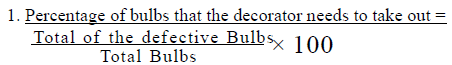• (Total number of bulbs) = (Number of Bulbs in set A) + (Number of Bulbs in set B)
• (Total number of defective bulbs) = (Number of defective bulbs in set A) + (Number of defective bulbs in set B)

Working out:

1. Total bulbs = 100 + 80 = 180
2. Total defective bulbs = 20 + 40 = 60
3. Minimum percentage of bulbs that he needs to take out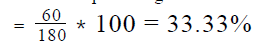4. So, the decorator should take out at-least 34% of the light bulbs

QUESTION: 16

Abe and Beth both start from a common point and travel in different directions towards their respective destinations. Abe’s
average speed is 25% greater than Beth’s average speed but Abe needs to cover 50% greater distance than Beth. Which of the
following is closest to the percentage by which the travel time of Beth lesser than that of Abe?

Solution:

Given:

• Let Abe’s Average Speed, Distance covered and Time taken be S , D and T respectively
• Let Beth’s Average Speed, Distance covered and Time taken be S , D and T respectively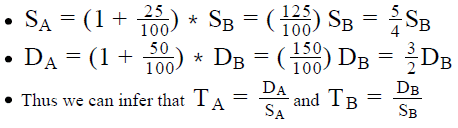To Find: approx. % by which TB is lesser than

Approach:

1. % by which T is lesser than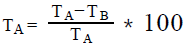2. So, to answer the question, we need to find:
• Either the values of TA and TB
• Or TA in terms of TB
3. Using the given relations between S and S , and between DA and DB , we’ll express T in terms of T and hence, answer the question

Working out: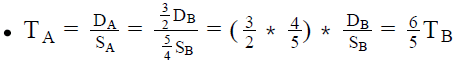• So, % by which TA is lesser than TB =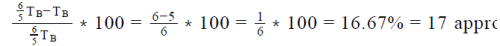Looking at the answer choices, we see that Option A is correct

QUESTION: 17

Five friends Adi, Brian, Close, Derek and Eli appeared in two aptitude tests. In the first aptitude test, Derek’s score was 50 percent less than the average (arithmetic mean) score of the five people. In the second aptitude test, Derek scored 50 percent more than what he scored on the first aptitude test. If the scores of his friends in the second aptitude test were same as their scores in the first aptitude test, by approximately what percentage was Derek’s score less than the average (arithmetic mean) score of the 5 people in the second aptitude test?

Solution:

Given:

1. 1st Aptitude Test
• Let the scores of Adi, Brian, Close, Derek and Eli be a, b, c, d and e respectively
• Let the average score be x
• d = x – 50% of x = 0.5x
2. 2nd Aptitude Test
• Adi, Brian, Close and Eli scored a, b, c and e respectively
• Derek’s score = d + 50% of d = 1.5d
• Let the average score be y

To Find: By approximately what percentage was Derek’s score less than the average (arithmetic mean) score of the 5 people in the second aptitude test?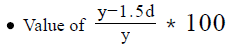Approach:

We can see from the expression above that if we could express y or d in terms of a common variable, we will be able to calculate our answer

1. Expression of y: As y is the average score of the 2 aptitude test, we can express y in terms of a, b, c, d and e.
2. Expression of x: Also, in the 1 aptitude test, as x is the average score, we can express x in terms of a, b, c, d, and e.
• Using (2) and (3), we can express y in terms of x and d( We cannot express y solely in terms of x, because in, the coefficient of d is different to the coefficient of d in x.)
3. Expression of d : Using the relation “Derek’s score was 50 percent less than the average (arithmetic mean) score of the five people “ in the 1 aptitude test, we can also express d in terms of x.
4. Using (2.a) and (3), we can express y in terms of x only.
5. As in (3), we have already expressed d in terms of x only, we have achieved our objective of expressing y and d in terms of a common variable i.e. x, which will give us the answer

Working out:

1. Expression of y: Average score in the 2nd aptitude test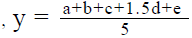2. Expression of x: Average score in the 1st aptitude test
• Using (1) and (2), we can
•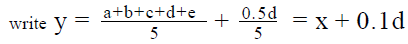3. ​Expression of d: As “Derek’s score was 50 percent less than the average (arithmetic mean) score of the five people” in the 1 aptitude test, we can write d = 0.5x
4.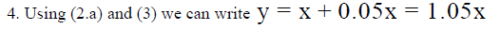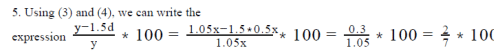QUESTION: 18

if A = x% of y and B = y% of x, then which of the following is true?

Solution: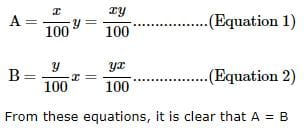QUESTION: 19

Two employees X and Y are paid a total of Rs. 550 per week by their employer. If X is paid 120 percent of the sum paid to Y, how much is Y paid per week?

Solution:

Let the amount paid to X per week = x
and the amount paid to Y per week = y
Then x + y = 550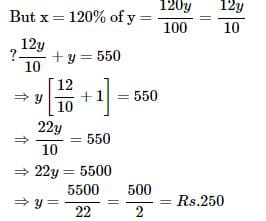QUESTION: 20

In a competitive examination in State A, 6% candidates got selected from the total appeared candidates. State B had an equal number of candidates appeared and 7% candidates got selected with 80 more candidates got selected than A. What was the number of candidates appeared from each State?

Solution:

State A and State B had an equal number of candidates appeared.
In state A, 6% candidates got selected from the total appeared candidates
In state B, 7% candidates got selected from the total appeared candidates
But in State B, 80 more candidates got selected than State A
From these, it is clear that 1% of the total appeared candidates in State B = 80
=> total appeared candidates in State B = 80 x 100 = 8000
=> total appeared candidates in State A = total appeared candidates in State B = 8000Use Code STAYHOME200 and get INR 200 additional OFF Use Coupon Code